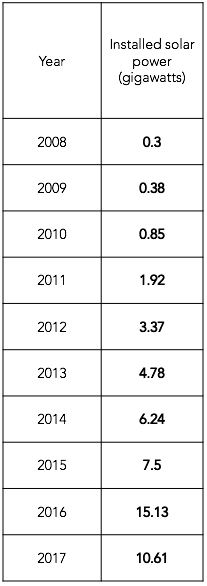# SOLAR ENERGY IN THE US

Skills: Addition & Subtraction (Decimal numbers)

Related environmental issues: Energy /  Renewable Energy

The table below shows shows how much new solar power was installed the United States each year between 2008 and 2017. Solar power means electricity generated by solar panels.

1 Gigawatt = 100,000,000 kilowatts (With 1 gigawatt of solar power, we can power 190,000 homes!)1. Which year has the largest number?

2. What is the difference between the numbers of the years 2014 and 2015?

3. Is the amount of solar panels installed in 2011 more than those installed in 2009 and 2010 combined or less?

4. What is the difference between the largest number and the smallest number?

5. Which year has the number 4.4 larger than  0.38?

6. Which two numbers make the largest value when added?

Sources:

__________________________________________________________